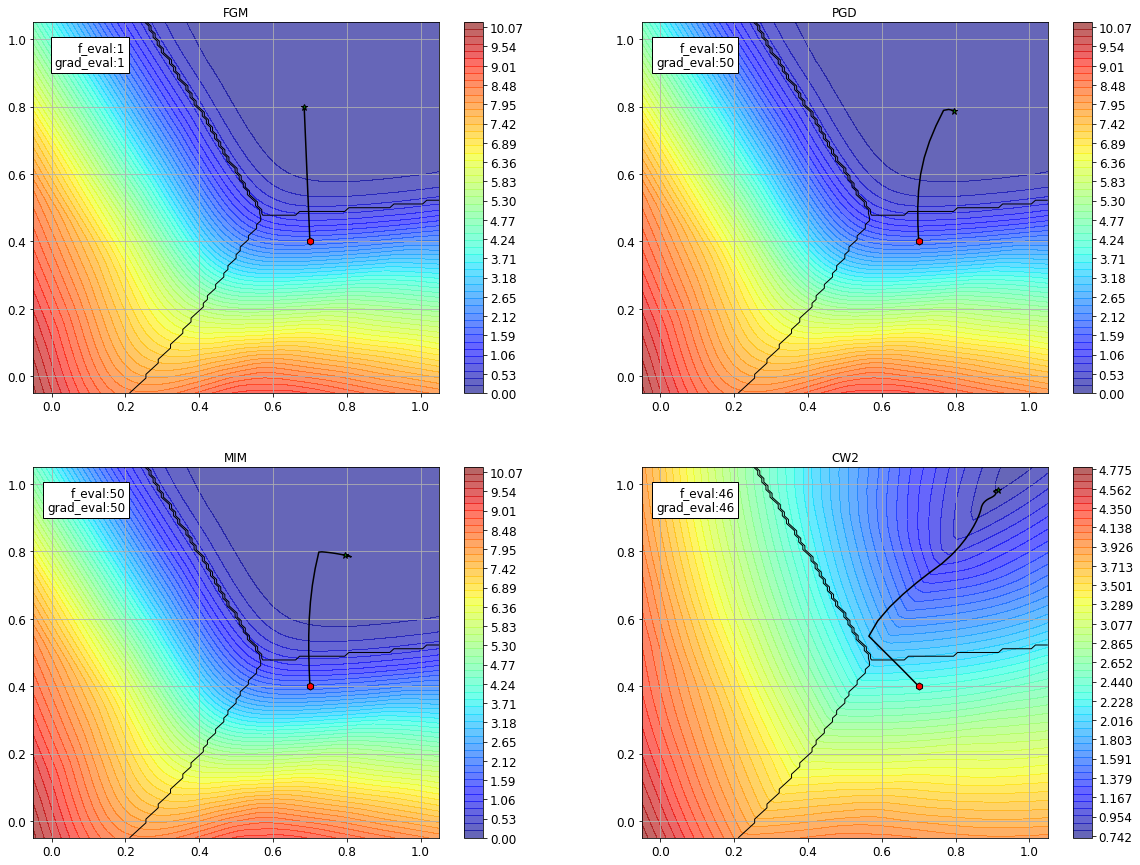# 2.8. Using cleverhans within SecML¶

In this tutorial we will show how to craft evasion attacks against machine learning models in SecML through the Cleverhans interface.Warning

Requires installation of the pytorch and cleverhans extra dependencies. See extra components for more information.

:

%%capture --no-stderr --no-display
# NBVAL_IGNORE_OUTPUT

try:
import secml
import torch
import cleverhans
except ImportError:
%pip install git+https://gitlab.com/secml/secml#egg=secml[pytorch,cleverhans]


## 2.8.1. Training the model¶

The first part is the same as the (first notebook). We load here a 2D dataset, so that we can easily plot the attack initial point and path.

:

random_state = 999

n_features = 2  # Number of features
n_samples = 1100  # Number of samples
centers = [[-2, 0], [2, -2], [2, 2]]  # Centers of the clusters
cluster_std = 0.75  # Standard deviation of the clusters
n_classes = len(centers)

dataset = CDLRandomBlobs(n_features=n_features,
centers=centers,
cluster_std=cluster_std,
n_samples=n_samples,

n_tr = 1000  # Number of training set samples
n_ts = 100  # Number of test set samples

# Split in training and test
from secml.data.splitter import CTrainTestSplit

splitter = CTrainTestSplit(
train_size=n_tr, test_size=n_ts, random_state=random_state)
tr, ts = splitter.split(dataset)

# Normalize the data
from secml.ml.features import CNormalizerMinMax

nmz = CNormalizerMinMax()
tr.X = nmz.fit_transform(tr.X)
ts.X = nmz.transform(ts.X)

# Metric to use for training and performance evaluation
from secml.ml.peval.metrics import CMetricAccuracy

metric = CMetricAccuracy()

# Creation of the multiclass classifier
import torch
from torch import nn

class Net(nn.Module):
"""
Model with input size (-1, 5) for blobs dataset
with 5 features
"""
def __init__(self, n_features, n_classes):
"""Example network."""
super(Net, self).__init__()
self.fc1 = nn.Linear(n_features, 50)
self.fc2 = nn.Linear(50, n_classes)

def forward(self, x):
x = torch.relu(self.fc1(x))
x = self.fc2(x)
return x

# Random seed for PyTorch
torch.manual_seed(random_state)

# torch model creation
net = Net(n_features=n_features, n_classes=n_classes)

from torch import optim
criterion = nn.CrossEntropyLoss()
optimizer = optim.SGD(net.parameters(),
lr=0.001, momentum=0.9)

# wrap torch model in CClassifierPyTorch class
from secml.ml.classifiers import CClassifierPyTorch
clf = CClassifierPyTorch(model=net,
loss=criterion,
optimizer=optimizer,
input_shape=(n_features,),
epochs=5,
random_state=random_state)

# We can now fit the classifier
clf.fit(tr.X, tr.Y)

# Compute predictions on a test set
y_pred = clf.predict(ts.X)

# Evaluate the accuracy of the classifier
acc = metric.performance_score(y_true=ts.Y, y_pred=y_pred)

print("Accuracy on test set: {:.2%}".format(acc))

Accuracy on test set: 100.00%


## 2.8.2. Preparing the attacks¶

Now that we have the model we can prepare the attacks. We will test several attack algorithm from cleverhans library.

We can specify a starting point for the attacks, we select a point from the class 1, which is in the lower right-corner of the 2D plane. As always, we can define a box for the attack in order to comply with the feature range ([0, 1]) and a maximum distance, as well as the target class (as always, specifying y_target=None will produce an untargeted attack).

:

from secml.array import CArray

# x0, y0 = ts[5, :].X, ts[5, :].Y  # Initial sample
x0, y0 = CArray([0.7, 0.4]), CArray()
lb, ub = 0, 1
dmax = 0.4
y_target = 2


We can finally specify the parameters for the attacks. We can compare the paths of several attacks, for which the parameters can be found in cleverhans docs.

We are going to use the following attacks:

• FGM Goodfellow IJ, Shlens J, Szegedy C. Explaining and Harnessing Adversarial Examples. arXiv:14126572 [cs, stat] [Internet]. 2014

• PGD Kurakin A, Goodfellow I, Bengio S. Adversarial examples in the physical world. arXiv:160702533 [cs, stat] [Internet]. 2017

• MIM Dong Y, Liao F, Pang T, Su H, Zhu J, Hu X, et al. Boosting Adversarial Attacks with Momentum. In: 2018 IEEE/CVF Conference on Computer Vision and Pattern Recognition [Internet]. Salt Lake City, UT: IEEE; 2018

• CW Carlini N, Wagner D. Towards Evaluating the Robustness of Neural Networks. arXiv:160804644 [cs] [Internet]. 2016

:

from cleverhans.attacks import CarliniWagnerL2, ProjectedGradientDescent, \

from collections import namedtuple
Attack = namedtuple('Attack', 'attack_cls short_name attack_params')

attacks = [
'clip_max': ub,
'clip_min': lb,
'ord': 2}),
'eps_iter': 0.05,
'nb_iter': 50,
'clip_max': ub,
'clip_min': lb,
'ord': 2,
'rand_init': False}),
Attack(MomentumIterativeMethod, 'MIM', {'eps': dmax,
'eps_iter': 0.05,
'nb_iter': 50,
'clip_max': ub,
'clip_min': lb,
'ord': 2,
'decay_factor': 1}),
Attack(CarliniWagnerL2, 'CW2', {'binary_search_steps': 1,
'initial_const': 0.2,
'confidence': 10,
'abort_early': True,
'clip_min': lb,
'clip_max': ub,
'max_iterations': 50,
'learning_rate': 0.1})]


## 2.8.3. Running the attacks¶

We can now run the attacks by passing them to the CAttackEvasionCleverhans class, which handles the attack optimization and provides useful output as the attack path and objective function. We can plot these information with the help of the CFigure module and its powerful APIs plot_function and plot_path.

:

from secml.figure import CFigure
# Only required for visualization in notebooks
%matplotlib inline

fig = CFigure(width=20, height=15)

for i, attack in enumerate(attacks):
fig.subplot(2, 2, i + 1)

fig.sp.plot_decision_regions(clf,
plot_background=False,
n_grid_points=100)

cleverhans_attack = CAttackEvasionCleverhans(
classifier=clf,
y_target=y_target,
clvh_attack_class=attack.attack_cls,
**attack.attack_params)

# Run the evasion attack on x0
print("Attack {:} started...".format(attack.short_name))
y_pred_CH, _, adv_ds_CH, _ = cleverhans_attack.run(x0, y0)
print("Attack finished!")

fig.sp.plot_fun(cleverhans_attack.objective_function,
multipoint=True, plot_levels=False,
n_grid_points=50, alpha=0.6)

print("Original x0 label: ", y0.item())
"".format(attack.attack_cls.__name__), y_pred_CH.item())

print("Number of classifier function evaluations: {:}"
"".format(cleverhans_attack.f_eval))
print("Number of classifier gradient evaluations: {:}"

fig.sp.plot_path(cleverhans_attack.x_seq)
fig.sp.title(attack.short_name)
"".format(cleverhans_attack.f_eval,
bbox=dict(facecolor='white'), horizontalalignment='right')
fig.show()

Attack FGM started...
Attack finished!
Original x0 label:  1
Number of classifier function evaluations: 1
Number of classifier gradient evaluations: 1
Attack PGD started...
Attack finished!
Original x0 label:  1
Number of classifier function evaluations: 50
Number of classifier gradient evaluations: 50
Attack MIM started...
Attack finished!
Original x0 label:  1